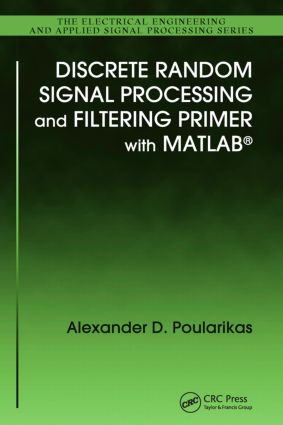# Discrete Random Signal Processing and Filtering Primer with MATLAB

## 1st Edition

CRC Press

304 pages | 110 B/W Illus.

Hardback: 9781420089332
pub: 2008-11-24
SAVE ~\$19.39
\$96.95
\$77.56
x
eBook (VitalSource) : 9781315218564
pub: 2018-10-03
from \$48.48

FREE Standard Shipping!

### Description

Engineers in all fields will appreciate a practical guide that combines several new effective MATLAB® problem-solving approaches and the very latest in discrete random signal processing and filtering.

Numerous Useful Examples, Problems, and Solutions – An Extensive and Powerful Review

Written for practicing engineers seeking to strengthen their practical grasp of random signal processing, Discrete Random Signal Processing and Filtering Primer with MATLAB provides the opportunity to doubly enhance their skills. The author, a leading expert in the field of electrical and computer engineering, offers a solid review of recent developments in discrete signal processing. The book also details the latest progress in the revolutionary MATLAB language.

A Practical Self-Tutorial That Transcends Theory

The author introduces an incremental discussion of signal processing and filtering, and presents several new methods that can be used for a more dynamic analysis of random digital signals with both linear and non-linear filtering. Ideal as a self-tutorial, this book includes numerous examples and functions, which can be used to select parameters, perform simulations, and analyze results. This concise guide encourages readers to use MATLAB functions – and those new ones introduced as Book MATLAB Functions – to substitute many different combinations of parameters, giving them a firm grasp of how much each parameter affects results.

Much more than a simple review of theory, this book emphasizes problem solving and result analysis, enabling readers to take a hands-on approach to advance their own understanding of MATLAB and the way it is used within signal processing and filtering.

Fourier analysis of signals

Introduction

FT

Sampling of signals

Discrete-time FT (DTFT)

DFT

Resolution

Continuous linear systems

Discrete systems—linear difference equations

Detrending

Random variables, sequences, and stochastic processes

Random signals and distributions

Averages

Stationary processes

Wiener–Khinchin relations

Filtering random processes

PDFs

Estimators

Confidence intervals

Nonparametric (classical) spectrums estimation

Periodogram and correlogram spectra estimators

Daniell periodogram

Bartlett periodogram

Blackman–Tukey (BT) method

Welch method

Proposed modified methods for Welch periodogram

Parametric and other methods for spectra estimation

AR, MA, and ARMA models

Yule–Walker (YW) equations

Least-squares (LS) method and linear prediction

Minimum variance (MV) method

Model order

Levinson–Durbin algorithm

Maximum entropy method

Spectrums of segmented signals

Eigenvalues and eigenvectors of matrices

Optimal filtering—Wiener filters

Mean square error (MSE)

FIR Wiener filter

Wiener solution—orthogonal principle

Wiener filtering examples

Introduction

LMS algorithm

Examples using the LMS algorithm

Properties of the LMS method

Adaptive filtering with variations of LMS algorithm.

Sign algorithms

Normalized LMS (NLMS) algorithm

Variable step-size LMS algorithm (VSLMS)

Leaky LMS algorithm

Linearly constrained LMS algorithm

Convergence in transform domain of the adaptive LMS filtering

Error-normalized LMS algorithm

Nonlinear filtering

Introduction

Statistical preliminaries

Mean filter

Median filter

Trimmed-type mean filter

L-filters

Ranked-order statistic filter

Edge-enhancement filters

R-filters

Appendix A: Suggestions and explanations for MATLAB® use

Appendix B: Matrix analysis

Appendix C: Lagrange multiplier method